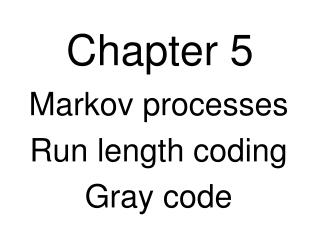DownloadDownload PresentationChapter 5

# Chapter 5

Télécharger la présentation## Chapter 5

- - - - - - - - - - - - - - - - - - - - - - - - - - - E N D - - - - - - - - - - - - - - - - - - - - - - - - - - -
##### Presentation Transcript

1. Chapter 5 Markov processes Run length coding Gray code

2. Markov Processes Transition Graph Transition Matrix Weather Example: Let (j = 1). Think: a means “fair” b means “rain” c means “snow” ½ next symbol b ⅓ ⅓ c u r r e n t s t a t e ¼ ⅓ ⅓ ⅓ ¼ ½ ¼ ¼ ¼ ½ Let S = {s1, …, sq} be a set of symbols. A jth-order Markov process has probabilities p(si | si1 … sij) associated with it, the conditional probability of seeing si after seeing si1… sij. This is said to be a j-memory source, and there are qjstates in the Markov process. a ¼ ¼ = M ¼ c ⅓ ½ p(si | sj) p(si | sj) i = column, j = row sj si | transition probability ∑ outgoing edges = 1 5.2

3. Ergodic Equilibriums Definition: A Markov process M is said to be ergodic if • From any state we can eventually get to any other state. • The system reaches a limiting distribution. 5.2

4. Predictive Coding Assume a prediction algorithm for the source which given all prior symbols, predicts the next. input stream prediction s1 ….. sn1pnen = pnsn error What is transmitted is the error, ei. By knowing just the error, the predictor also knows the original symbols. en en   sn sn destination channel source pn pn predictor predictor must assume that both predictors are identical, and start in the same state 5.7

5. Accuracy: The probability of the predictor being correct is p = 1  q; constant (over time) and independent of other prediction errors. Let the probability of a run of exactly n 0’s, (0n1), be p(n) = pn ∙ q. The probability of runs of any length n = 0, 1, 2, … is: Note: alternate method for calculating f(p), look at 5.8

6. Coding of Run Lengths Send a k-digit binary number to represent a run of zeroes whose length is between 0 and 2k 2. (small runs are in binary) For run lengths larger than 2k 2, send 2k 1 (k ones) followed by another k-digit binary number, etc. (large runs are in unary) Let n = run length. Fix k = block length. Let n = i ∙ m + j 0 ≤ j < m = 2k 1 like “reading” the “matrix” with m cells and ∞ many rows. 5.9

7. Expected length of run length code Let p(n) = the probability of a run of exactly n 0’s: 0n1. The expected code length is: But every n can be written uniquely as i∙m + j where i ≥ 0, 0 ≤ j < m = 2k 1. 5.9

8. Gray Code Consider an analog-to-digital “flash” converter consisting of a rotating wheel: 0 0 The maximum error in the scheme is ± ⅛ rotation because … 1 0 imagine “brushes” contacting the wheel in each of the three circles 0 0 0 1 1 1 0 0 0 0 0 1 1 0 1 1 1 1 0 1 1 The Hamming Distance between adjacent positions is 1. In ordinary binary, the maximum distance is 3 (the max. possible). 5.15-17

9. 1-bit Gray code 5.15-17